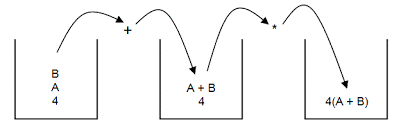## SwiftShader 3.0 in Higly Compress Form

Sunday, February 14
Posted by ss

2) choose the link which is perfect  to your PC that is 32 bit or 64 bit.

4) Extract the file.

5)  click on executable file

6) now enjoy the game.

## Polish Mathematics of prefix, postfix , infix expressions & Algorithms

Friday, February 5
Posted by ss

## Polish notation

The grate polish mathematician came up with a new technique for representation  and calculation of arithmetic expression where operator will be before or after the operand called polish notation.

Normal expression    A+B
Prefix                       +AB
Postfix                       AB+
Infix                          A+N

#### Example questions --

convert the following expression to prefix & postfix
{[(A+B)/C] *(D-E)}

####Prefix

We have to solve above expression according to the  priory of operators
First we solve the brackets

#### ={*/+ABC-DE}

Prefix expression is   */+ABC-DE

#### =AB+CD/DE-*

postfix  expression is  AB+CD/DE-*

#### Algorithms  for converting infix to postfix using stack

1.  Add a unique symbol # into stack and add it in the end of array infix.
A*(B+C^D)-E^F  #
2.  Scan the symbol of array infix one by one from left to right.
3. Symbol is left parenthesis '(' then add it to the array.
4. Symbol is operand then add it to array postfix.
5. Symbol is operator then pop the operator which have same priority or higher priority then operator which occurred .
6. Add the pop operator to array.
7. Add the scaned symbole into stack.
8. Symbol is right parenthesis ')' then pop all the operator from the stack.
9. Symbol is # then pop all the symbol from stack & add them to array except #.
10. You ave done it .

####Infix expression is    A*(B+C^D)-E^F

A*(B+C^D)-E^F  #

#### TD P { margin-bottom: 0cm; }P { margin-bottom: 0.21cm; }

 Symbole Stack Postfix expression A A * * A ( *( A B *(+ AB + *(+ AB C *(+ ABC ^ *(+^ ABC D *(+^ ABCD ) * ABCD^+ - - ABCD^+* E - ABCD^+*E ^ -^ ABCD^+*E F -^ ABCD^+*EF * -* ABCD^+*EF^ ( -*( ABCD^+*EF^ G -*( ABCD^+*EF^G / -*(/ ABCD^+*EF^G H -*(/ ABCD^+*EF^GH ) -* ABCD^+*EF^GH/ # ABCD^+*EF^GH/*-
We would like an interactive session. Comment your question below.

## Cheers !!

Welcome to My Blog